# Multiplying Decimals by Whole Numbers & Decimals Lesson Plan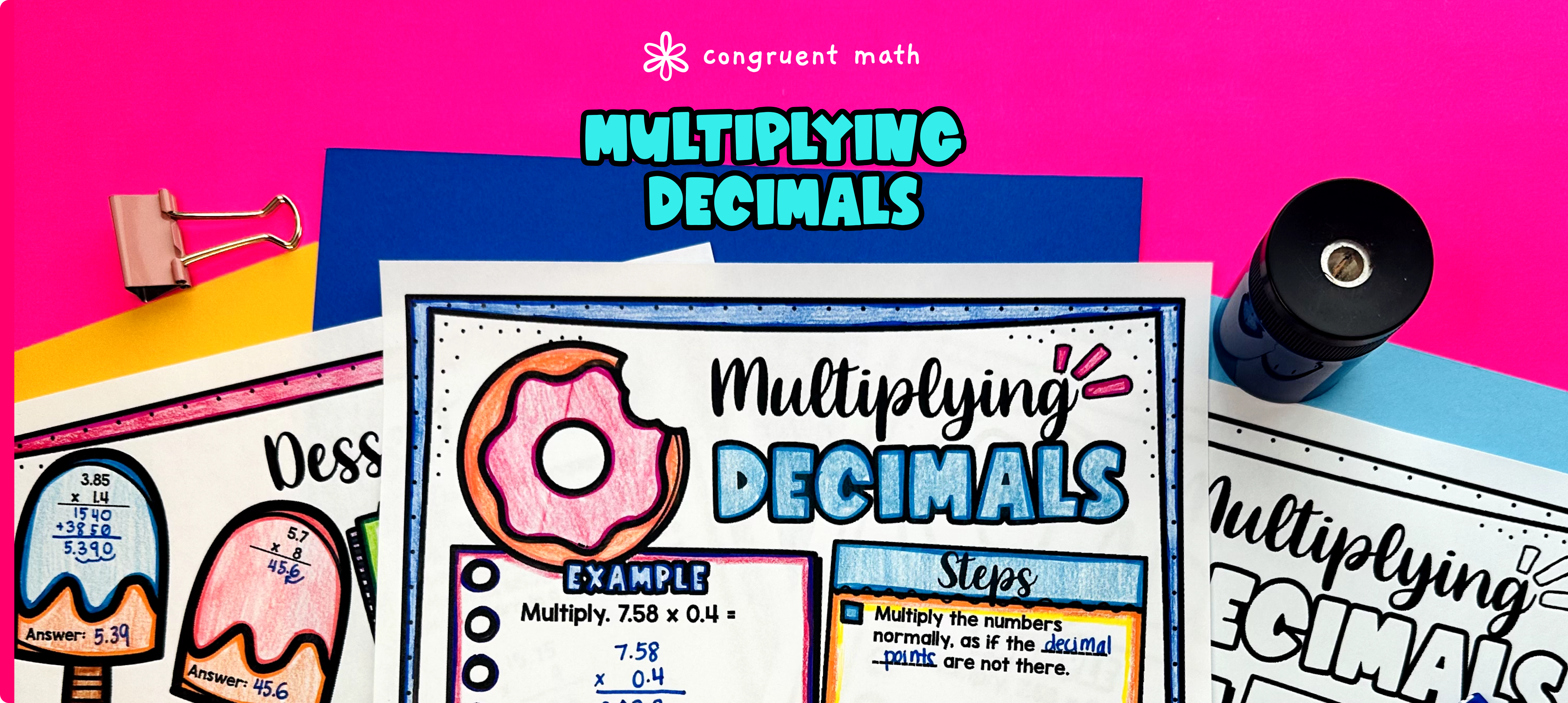Pin This

## Overview

Ever wondered how to teach multiplying decimals by whole numbers and decimals in an engaging way to your 5th and 6th grade math students?

In this lesson plan, students will learn about multiplying decimals by whole numbers and decimals and their real-life applications. Through artistic, interactive guided notes, check for understanding, a doodle & color by number activity, and a maze worksheet, students will gain a comprehensive understanding of multiplying decimals.

The lesson culminates with a real-life example that explores how multiplying decimals is applied to planning an international trip and currency conversions.

## Get the Lesson Materials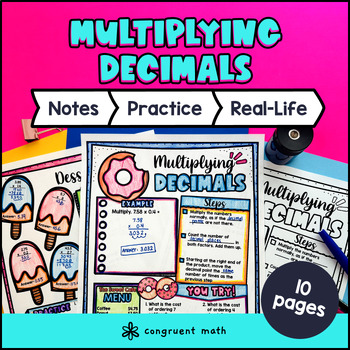\$4.25

## Learning Objectives

After this lesson, students will be able to:

• Multiply decimals by whole numbers

• Multiply decimals by decimals

• Apply the concept of multiplying decimals to a real-life scenario, such as planning an international trip and currency conversions

## Prerequisites

Before this lesson, students should be familiar with:

• Place value and the value of decimal digits

• Multiplication of whole numbers

## Key Vocabulary

• Multiplying decimals

• Whole numbers

• Decimal places

• Product

## Procedure

### Introduction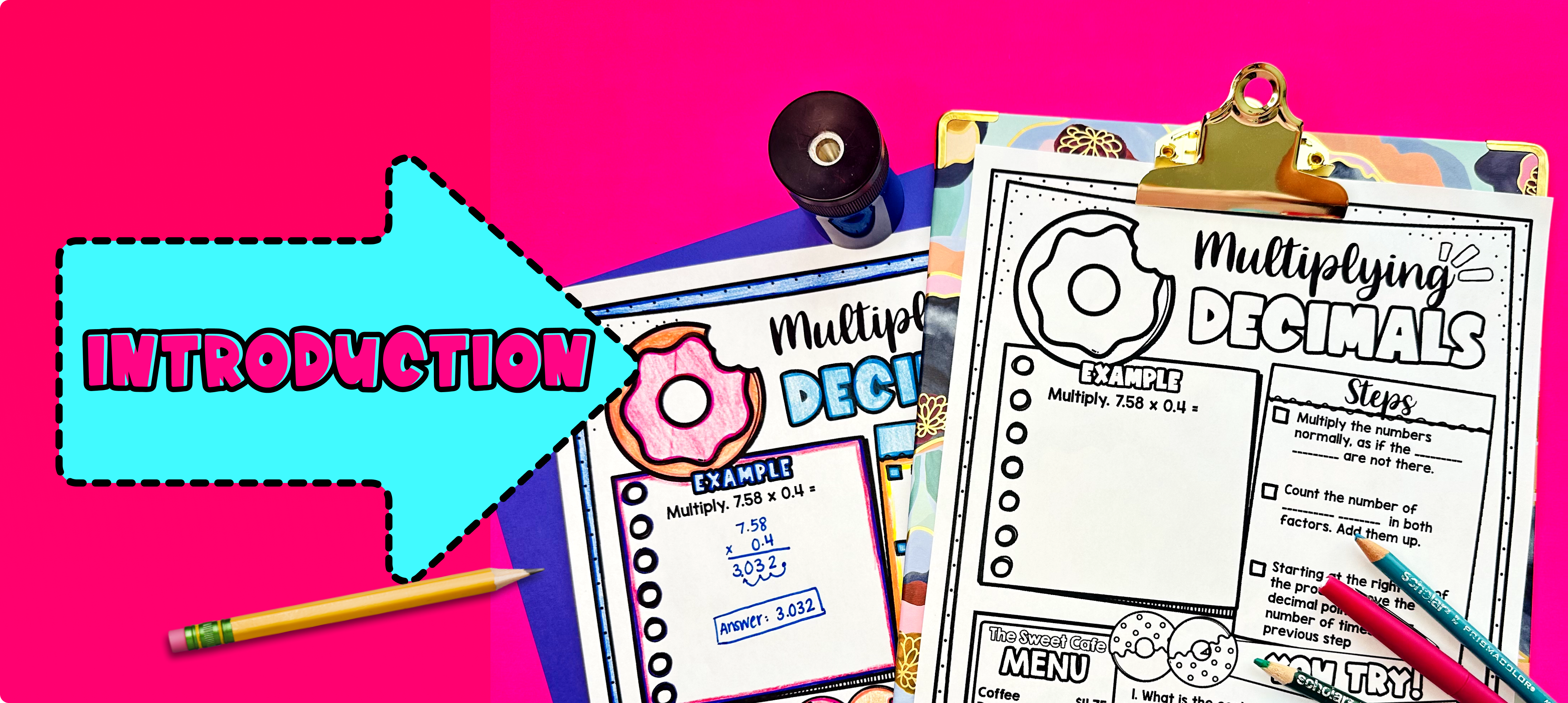Pin This

As a hook, ask students why multiplying decimals is important in real life situations. You can refer to the real-life application described on the last page of the guided notes, where students plan an international trip and make currency conversions. This will help engage students and set the context for learning about multiplying decimals.

Use the guided notes to introduce the topic of multiplying decimals by whole numbers and decimals. Walk through the key points of the topic, such as the steps involved in multiplying decimals and the concept of place value. Emphasize the importance of stacking decimal to the right when multiplying decimals (rather than lining up decimal place value as you would for decimal addition and subtraction). Also teach students how to count the number decimal place values in the factors to determine the placement of decimal point in the product. Walk through the example on the first page of the guided notes to ensure students understand each step. Refer to the FAQ section below for a walk through on this topic, as well as ideas on how to respond to common student questions.

Based on student responses, reteach concepts that students need extra help with. If your class has a wide range of proficiency levels, you can pull out students for reteaching, and have more advanced students begin work on the practice exercises.

### Practice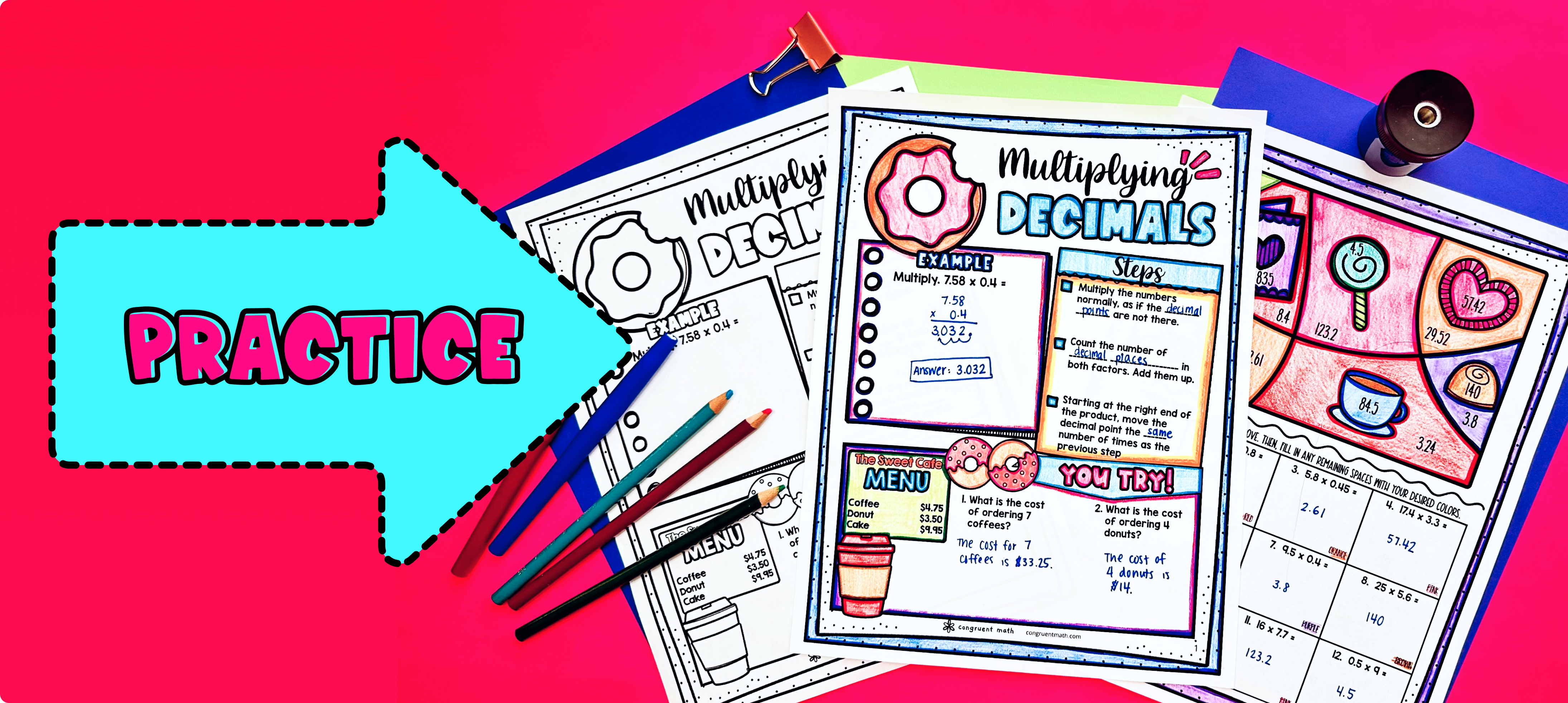Pin This

Have students practice multiplying decimals by whole numbers and decimals using the practice worksheets provided in the resource (page 2 of guided notes, called "Dessert Party). Students play the role of a customer buying different desserts and drinks in a cafe, using decimal multiplication to solve the problems. Walk around the classroom to answer any questions students may have while they work.

Fast finishers can engage in the maze activity or color by code activity, which offers extra practice. You may also assign it as homework for those who finish early.

### Real-Life Application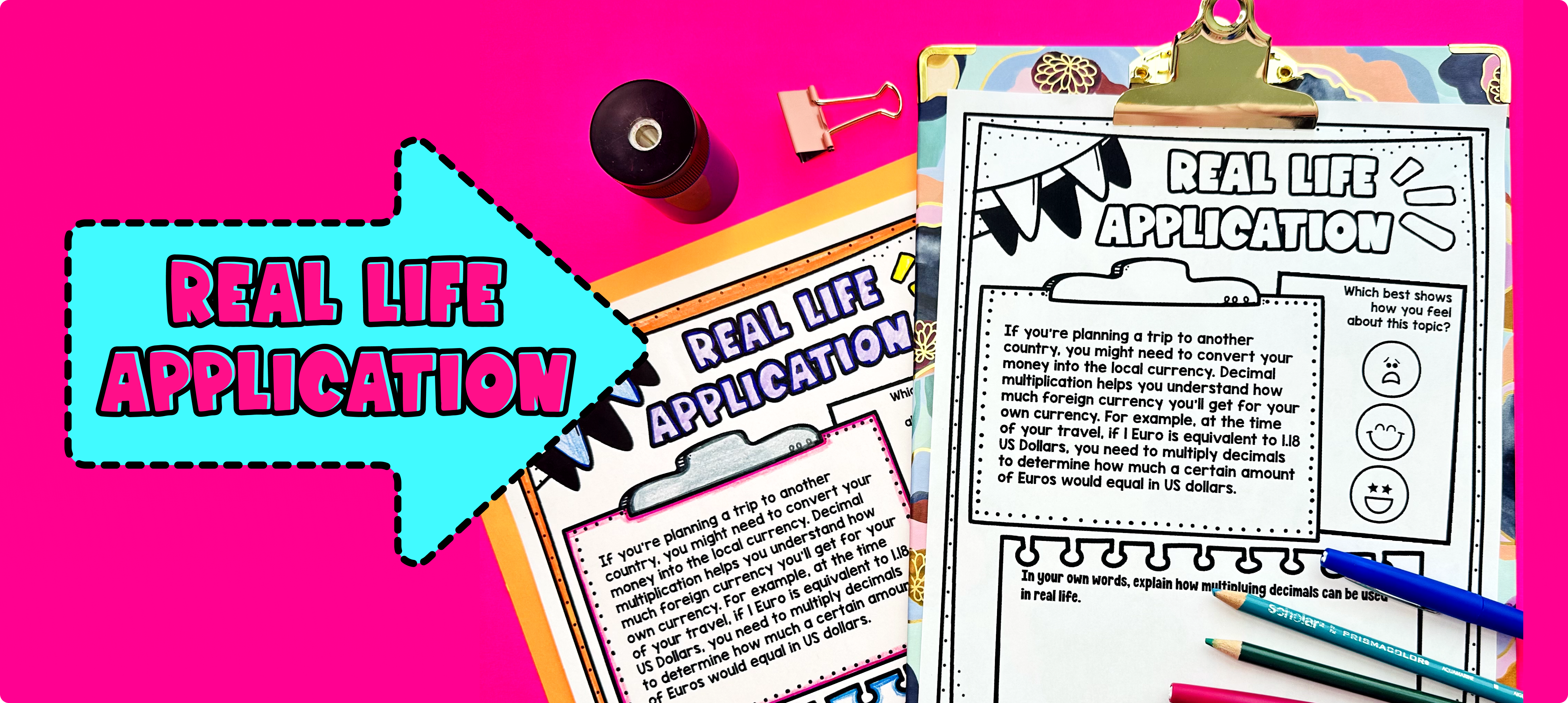Pin This

Bring the class back together, and introduce the concept of multiplying decimals as applied to planning an international trip and currency conversions. This is covered on the last page of the guided notes resource. Refer to the FAQ for more ideas on how to teach it!

## Extensions

If you’re looking for digital practice for multiplying decimals by decimals and whole numbers, try my Pixel Art activities in Google Sheets. Every answer is automatically checked, and correct answers unlock parts of a mystery picture. It’s incredibly fun, and a powerful tool for differentiation.

Here are some activities to explore:

A fun, no-prep way to practice multiplying decimals by decimals and whole numbers is Doodle Math — they’re a fresh take on color by number or color by code. It includes multiple levels of practice, perfect for a review day or sub plan.

Here is an activity to try:

### Real-Life Math Project

A fun way to wrap this lesson with your students is with one of my real-life math projects. They enable students to see the application of the math in an engaging, extended project:

## Want more ideas and freebies?

Get my free resource library with digital & print activities—plus tips over email.